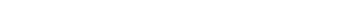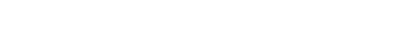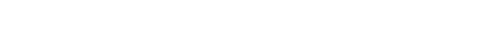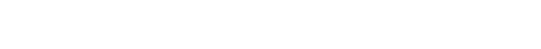# Chebyshev polynomials vs. Maclaurin ranks

In the comments to my article about the fast calculation of the sine, the question was asked: “Why didn’t the Taylor series expansion suit?”
The short answer is this: although an approximation using Taylor series (more precisely, Maclaurin series) gives a smaller error for the same number of calculations, it does not allow splitting the argument into an arbitrary number of intervals and thereby increasing the accuracy of calculations.

Now in more detail.

When approaching the sine (and not only) by Chebyshev polynomials, the following expression is used:In this case, the coefficients A0, A1 etc. calculated in advance for some part of the function.

Using Maclaurin series, the sine is calculated using the following formula:For the convenience of calculations, you can place the repeated fragments outside the parentheses. For example, like this:or like this:For example, let’s find the values ​​of the sine in the range from 0 toboth methods, and put their graphs together with the graph of the “real” sine. Take the Chebyshev polynomial of the 2nd degree (with three terms), and with the same number of terms take the Maclaurin series:

The graph of the Chebyshev polynomial is a fragment of a parabola, and intersects the graph of the sine at three points, which are the roots of the polynomial.
The graph of the Maclaurin series, starting from the left edge, almost coincides with the graph of the sine, and the discrepancy with it begins noticeably by eye only in the right third of the diagram.

The approximation error graphs look like this:

They show that the error of approximation by the Chebyshev polynomial is several times greater than that of the Maclaurin series.

Now let’s see how the approximation errors depend on the number of terms in the approximating function.
The graphs of approximation errors by Maclaurin series of different lengths look like this:

The graphs of the approximation errors by Chebyshev polynomials are as follows:

The error plots look different, but in both cases the error decreases with the increase in the number of members of the series. Moreover, the accuracy of the Chebyshev polynomial is already orders of magnitude worse than that of the Maclaurin series.

The following diagram shows the comparative accuracy of the approximation by both methods, depending on the number of terms in the series (polynomial). Precision is expressed in “fractional bits”, which are numerically equal to minus the logarithm of the base 2 error:

However, for approximation by Chebyshev polynomials, the full period (from 0 tocan be divided not into 4, as has been done so far, but, for example, into 512 equal intervals. This case is represented by a gray line in the diagram. The accuracy of 53.94 bits already with 6 terms (a polynomial of the 5th degree) exceeds that of the Maclaurin series of 51.62 bits with 10 terms of the series.